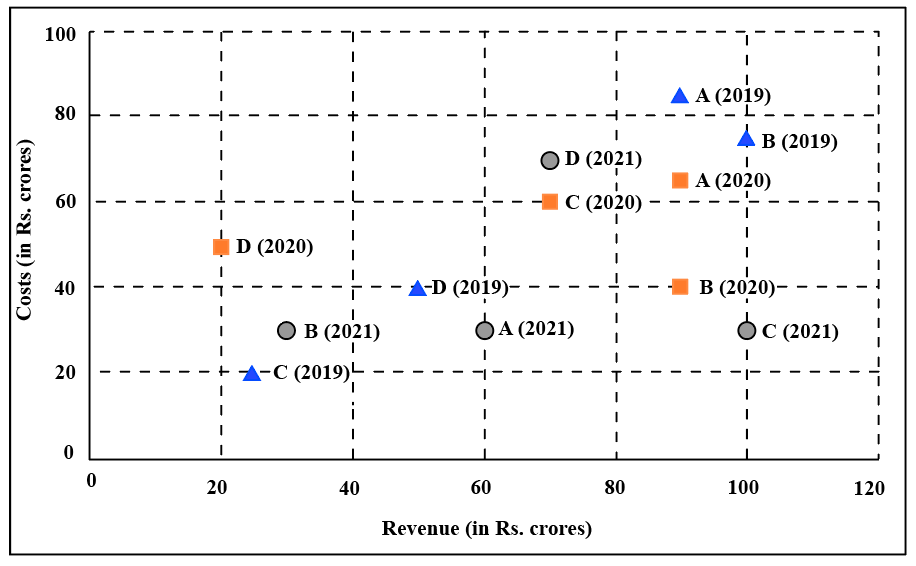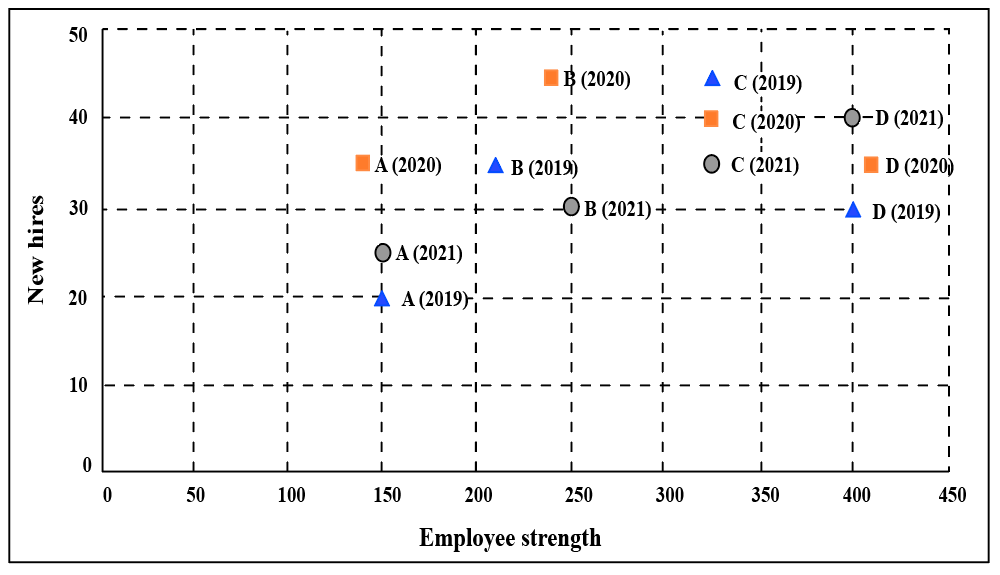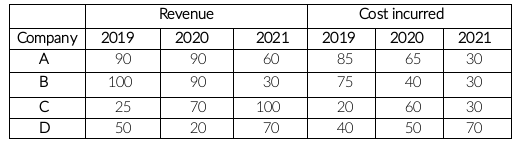### CAT 2022 Question Paper (Slot 2) Question 37

Instructions

The two plots below show data for four companies code-named A, B, C, and D over three years - 2019, 2020, and 2021.

The first plot shows the revenues and costs incurred by the companies during these years. For example, in 2021, company C earned Rs.100 crores in revenue and spent Rs.30 crores. The profit of a company is defined as its revenue minus its costsThe second plot shows the number of employees employed by the company (employee strength) at the start of each of these three years, as well as the number of new employees hired each year (new hires). For example, Company B had 250 employees at the start of 2021, and 30 new employees joined the company during the year.Question 37

# The ratio of a company's annual profit to its annual costs is a measure of its performance. Which of the four companies had the lowest value of this ratio in 2019?

Solution

It is given,Measure of A's performance in 2019 = $$\frac{90-85}{85}=\frac{5}{85}=0.06$$

Measure of B's performance in 2019 = $$\frac{100-75}{75}=\frac{25}{75}=0.33$$

Measure of C's performance in 2019 = $$\frac{25-20}{25}=\frac{5}{25}=0.2$$

Measure of D's performance in 2019 = $$\frac{50-40}{40}=\frac{10}{40}=0.25$$

Company A had the lowest value.